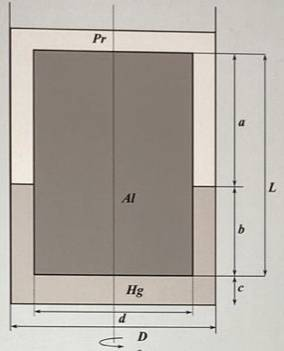# Total force that a fluid exerts on a cylinder

Guillem_dlc
Homework Statement:
A cylinder of diameter ##d=12,0\, \textrm{cm}## and height ##L=1,1\, \textrm{m}## is immersed floating at the interface between mercury (##\rho_{hg}=13580,0\, \textrm{kg}/\textrm{m}^3## and ##\mu_{hg}=0,0015\, \textrm{Pa}\cdot \textrm{s}##) and liquid paraffin (##\rho_{pr}=850,0\, \textrm{kg}/\textrm{m}^3## and ##\mu_{pr}=0,2\, \textrm{Pa}\cdot \textrm{s}##) within a glass tube of diameter ##D=12,2\, \textrm{cm}##. The cylinder is at ##c=0,2\, \textrm{cm}## from the bottom of the tube, the part immersed in mercury has a length of ##b=40,0\, \textrm{cm}## and the part immersed in liquid paraffin has a length of ##70,0\, \textrm{cm}##, as shown in the figure.

The cylinder is rotated by ##100,0 \, \textrm{rpm}##. Neglecting the friction at the base of the cylinder and the tube, determine the total force, in absolute value, that the fluid exerts on the cylinder, at ##\textrm{N}##.
Relevant Equations:
##F=\tau A##
Figure:I have calculated the density of the cylinder: ##5479,0\, \textrm{kg}/\textrm{m}^3##.

Attempt at a Solution:
$$d=0,12,\,\, L=1,1,\,\, D=0,122,\,\, e=0,002,\,\, c=0,02,\,\, b=0,4,\,\, a=0,7$$
$$\omega =100\, \textrm{rpm}=10,472\, \textrm{rad}/\textrm{s}\quad e=0,122-0,12=0,002$$
We know that: ##F=\tau A=\mu \dfrac{\omega r}{e}\cdot A\rightarrow##
We have two ##\mu##'s and two different areas:
• Hg ##\rightarrow A=\pi r^2+2\pi r\cdot b##
• Pr ##\rightarrow A=\pi r^2+2\pi ra##
$$\rightarrow F=\dfrac{\omega r}{e}(\pi r^2+2\pi rb+\pi r^2+2\pi ra)(\mu_{Hg}+\mu_{Pr})=$$
$$=\dfrac{\omega r}{e}(2\pi r^2+2\pi r(b+a))(\mu_{Hg}+\mu_{Pr})=\dfrac{\omega 2\pi r^2}{e}(1+b+a)(\mu_{Hg}+\mu_{Pr})$$
$$=50,11\, \textrm{N}$$
Here I don't know when I should use the integral and when I shouldn't. Would you do it like this?

•